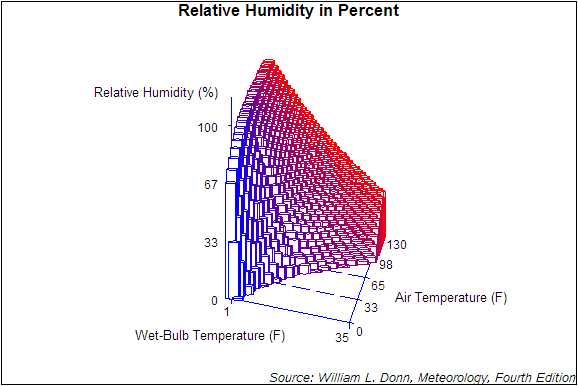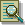The G3D Procedure

# Example 7: Generating a Rotated Scatter Plot with Modified Axes

Procedure features:
 Scatter statement CAXIS= COLOR= ROTATE= SHAPE= SIZE= XTICKNUM= YTICKNUM= ZTICKNUM= ZMAX= ZMIN=
Sample library member: GTDROTSCThis scatter plot modifies the procedure defaults to:

• specify a shape for the data points

• classify the data by color

• specify blue as the axis color

• rotates the X-Y plane -15 degrees around the perpendicular Z axis.

• specifies five major tick marks for the Y-axis

• specifies two major tick marks for the X-axis

• specifies five major tick marks for the Z-axis

• specifies the zero as the minimum axis value for the Z-axis

• specifies the one hundred as the maximum axis value for the Z-axis`goptions reset=all border;````title1 "Relative Humidity in Percent"; footnote1 j=r f="Albany ANT/it" "Source: William L. Donn, Meteorology, Fourth Edition";``````proc g3d data=sashelp.humid; scatter airtemp*bulbtemp=humidity/ shape=pillar color=colorvar caxis=blue rotate=-15 yticknum=5 xticknum=2 zticknum=4 zmin=0 zmax=100 run; quit;```Previous Page | Next Page | Top of Page